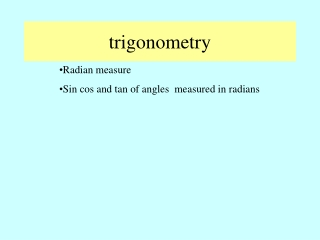Download Presentationtrigonometry

# trigonometry - PowerPoint PPT PresentationDownload Presentation## trigonometry

- - - - - - - - - - - - - - - - - - - - - - - - - - - E N D - - - - - - - - - - - - - - - - - - - - - - - - - - -
##### Presentation Transcript

1. trigonometry • Radian measure • Sin cos and tan of angles measured in radians

2. Radian measure A An angle is a measure of turn and so far we have measured angles in degrees 120º O < AOB = 120º The circle was divided into 360 sectors and the angle at the centre of each sector is 1º B 360 could have been chosen because it has many factors 1,2,3,4,5,6,8,9,10,12,15,18,20,24,30,36,40,45,60,72,90,120,180,360 The circle can then be divided into many sectors where the angle at the centre is a whole number So 360 was a convenient number to choose.

3. For more advanced maths the measure of turn must be a real number and not convenient number We therefore measure angles in radians The radian A r OA = OB = r the radius of the circle centre O r B When arc AB also = r r O Then angle AOB is measured to be 1 radian We can see that this is close to 60º Like an equilateral triangle

4. Comparing radians and degrees A r B = r O r = 1 radian =  radians = 180 º

5. We should now be able to change degrees into radians using 180 º =  radians 90 º = radians The fraction the angle 90 is of 180 is the same as the fraction the angle in radians is of  that is 1/2 Similarly 45º =  /4 60º = /3 30º = /6 Calculating angles > 90 Express as a multiple of 30, 45 or 60 135º = 3 X 45º = 3 X /4 =3/4 In most of our work with radians the angle is expressed as a multiple of  . So we should be able to convert easily between degrees and radians or memorise them

6. Copy ,complete and learn the following table changing degrees to radians

7. Changing degrees which are not multiples of 30,45 and 60to radians Change 70º to radians 180 º =  radians Start with Use direct proportion radians This is the exact value Using a calculator does not give the exact value 70º = 1.22 radians`

8. Change the following angles in degrees to radians give angle as a fraction of  and as a number to 3 dp degrees number fraction 1/9 0.349 20 º 40 º 80 º 140 º 200 º 320 º 144 º 280 º 0.698 2/9 1.396 4/9 2.443 7/9 3.840 11/9 5.59 16/9 2.513 4/5 14/9 4.887

9. Convert radians to degrees 3/2 = 3 X /2 = 3 X 90º = 270º 7/6 = 7 X /6 = 210º = 7 X 30º Other radians measures  Rads = 180º Using the calculator 343.8 º 6 rads = 6/ X 180 º

10. Change the following radian measures to degrees = 565º 7/X 180 7 rads 5/4 rads 6.28 rads 14 rads 7/12 rads 4.2 rads 1.4 rads 5/3 rads = 5 X 45º = 225º 5 X /4 6.28/ x180 = 360º 14/ x 180 = 802º = 105º = 7 X 15 7 X /12 4.2/ X 180 = 240.6º 1.4/ X180 = 97º 5 X /3 = 5 X 60 = 300º

11. Sin cos and tan of radian measures y = sin x 1 90º 360º degrees 180º 270º  3/2 Radians 2 /2 -1 When you draw graphs use radians on the x axis Ex 1 p 45 q 4

12. y = cos x 1 3/2 2 /2  -1

13. Trigonometry 60º 2 2 2 60º 60º 60º 2 3. Exact Values Some special values of Sin, Cos and Tan are useful left as fractions, We call these exact values Consider 30º 3 1 To provide values for 30º and 60º

14. 3 2 0 ½ 1 3 2 1 ½ 0 1 3 0 3

15. For 45º consider this square/ triangle 45º 2 1 1 45º 1 1

16. 3 2 1 2 0 ½ 1 3 2 1 2 1 ½ 1 3 0 1 3

17. The exact value of sin /3 = 3/2 = 0 The exact value of cos /2 We can also express these trig ratios to 2 decimal places using the calculator but remember to change to radian mode = 0 Cos /2 = cos (/2) Or cos 1.57 = 0 Ex 1 p 45 q 6,7 8